# How to Calculate and Solve for the Axis and Area of an Ellipse | Nickzom Calculator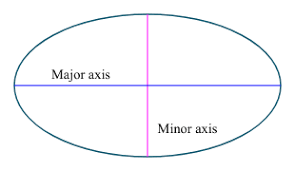The image above is an ellipse.

To compute the area of an ellipse, two essential parameters are needed and this parameters are axis (a) and axis (b).

A = πab

Where;
A = Area of the ellipse
a = Axis of the ellipse
b = Axis of the ellipse

Let’s solve an example;
Find the area of an ellipse when it has an axis (a) of 10 cm and an axis (b) of 17 cm.

This implies that;
a = Axis of the ellipse = 10 cm
b = Axis of the ellipse = 17 cm

A = πab
A = 3.142 x 10 x 17
A = 534.14

Therefore, the area of the ellipse is 534.14 cm2.

Calculating the Axis (a) of an ellipse using Area of the ellipse and Axis (b) of the ellipse.

a = A / πb

Where;
a = Axis of the ellipse
A = Area of the ellipse
b = Axis of the ellipse

Let’s solve an example;
Find the axis (a) of an ellipse when the area of the ellipse is 210 cm2 with an axis (b) of 19 cm.

This implies that;
A = Area of the ellipse = 210 cm2
b = Axis of the ellipse = 19 cm

a = A / πb
a = 210 / 3.142 x 19
a = 210 / 59.70
a = 3.52

Therefore, the axis (a) of an ellipse is 3.52 cm.

Calculating the Axis (b) of an ellipse using the Area of the ellipse and Axis (a) of the ellipse.

b = A / πa

Where;
b = Axis of the ellipse
A = Area of the ellipse
a = Axis of the ellipse

Let’s solve an example;
Find the axis (b) of an ellipse with an area of 320 cm2 and an axis (a) of 120 cm.

This implies that;
A = Area of the ellipse = 320 cm2
a = Axis of the ellipse = 120 cm

b = A / πa
b = 320 / 3.142 x 120
b = 320 / 377.04
b = 0.85

Therefore, the axis (b) of the ellipse is 0.85 cm.

Nickzom Calculator – The Calculator Encyclopedia is capable of calculating the area of an ellipse.

To get the answer and workings of the area of a ellipse using the Nickzom Calculator – The Calculator Encyclopedia. First, you need to obtain the app.

You can get this app via any of these means:

To get access to the professional version via web, you need to register and subscribe for NGN 1,500 per annum to have utter access to all functionalities.
You can also try the demo version via https://www.nickzom.org/calculator

Once, you have obtained the calculator encyclopedia app, proceed to the Calculator Map, then click on Mensuration under the Mathematics section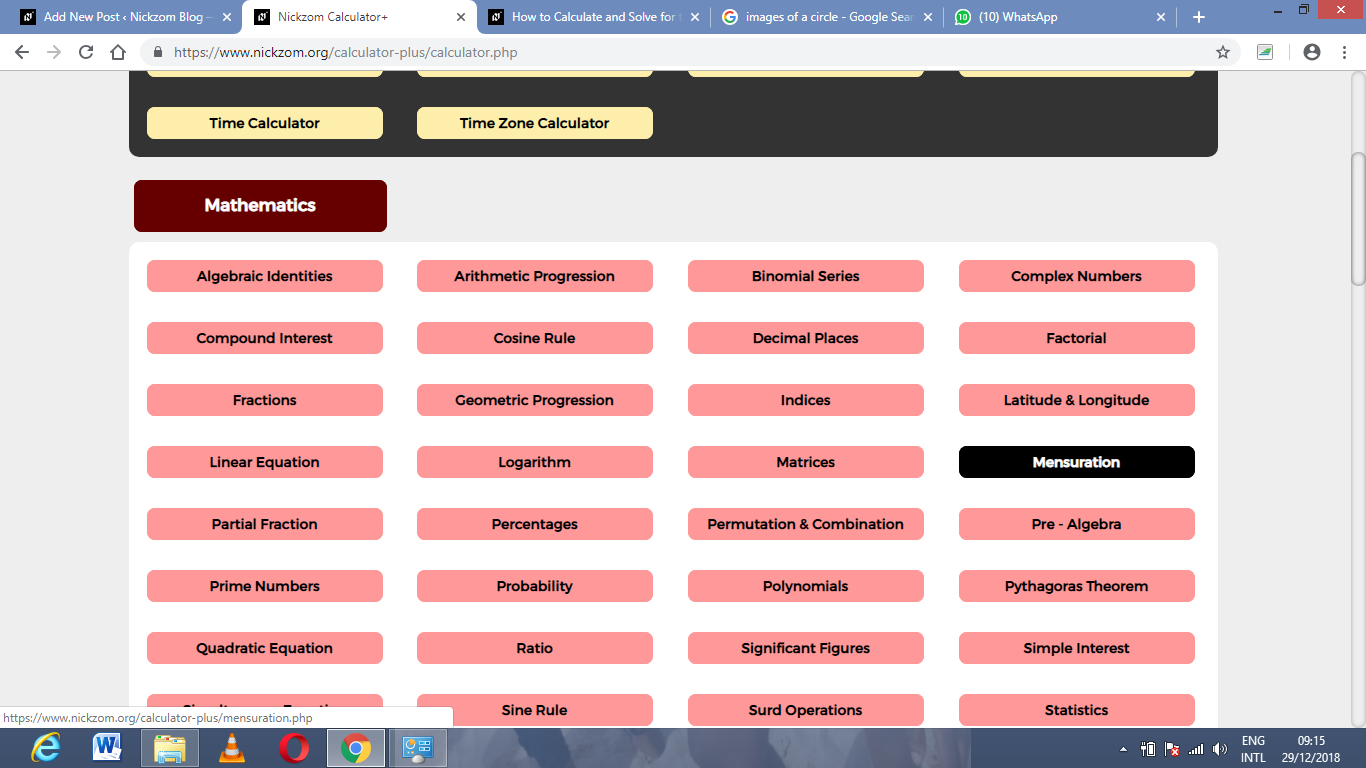Now, click on Area of an Ellipse under MensurationThe screenshot below displays the page or activity to enter your values, to get the answer for the area of an ellipse according to the respective parameters which are the axis (a) and axis (b) of the ellipse.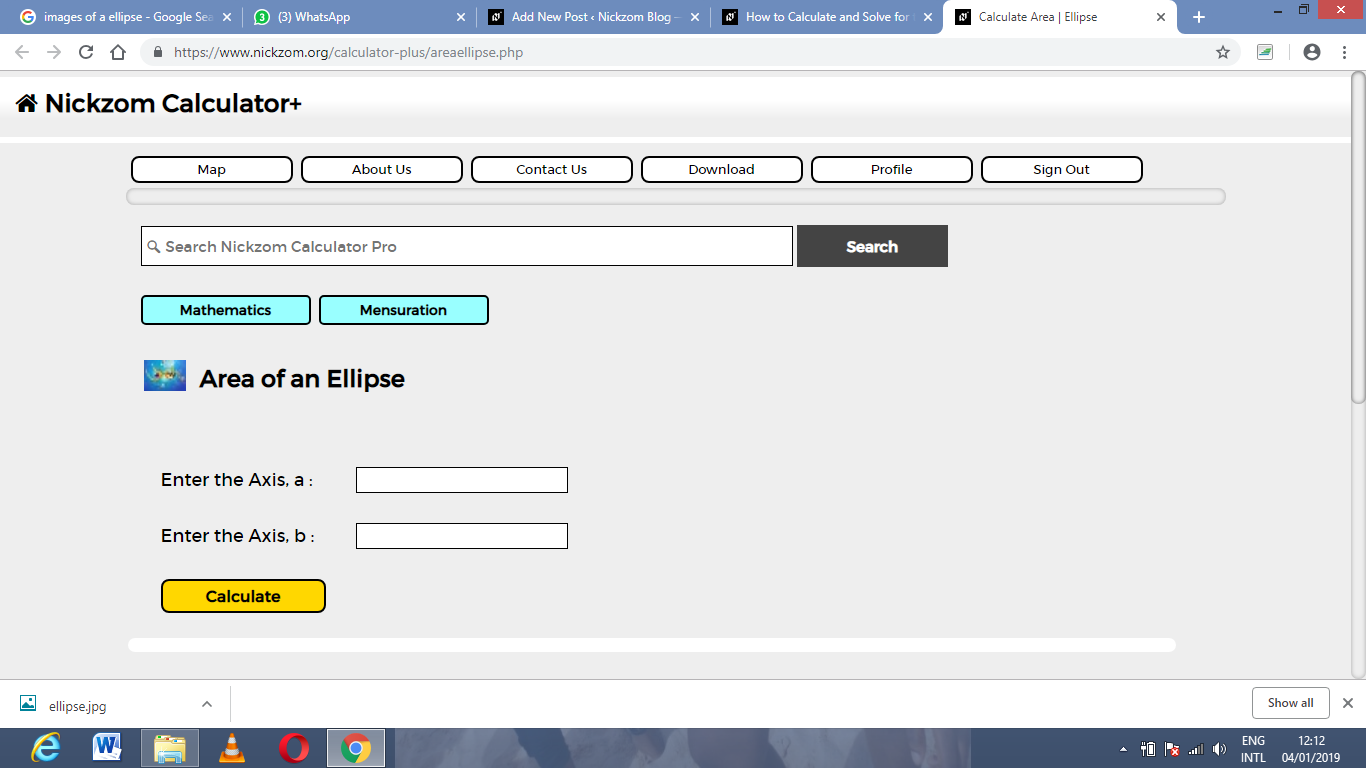Now, enter the values appropriately and accordingly for the parameters as required by the example above where the axis (a) is 10 cm and axis (b) is 17 cm.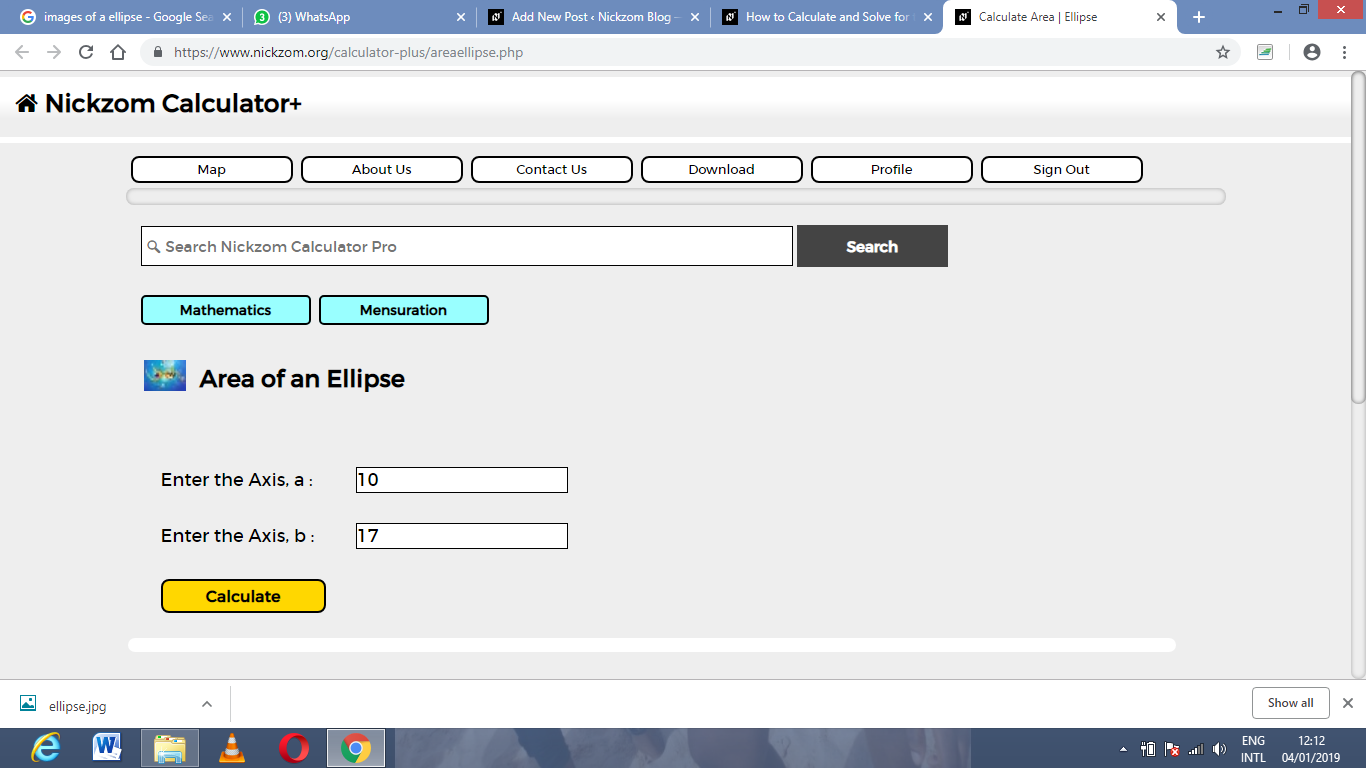Finally, Click on Calculate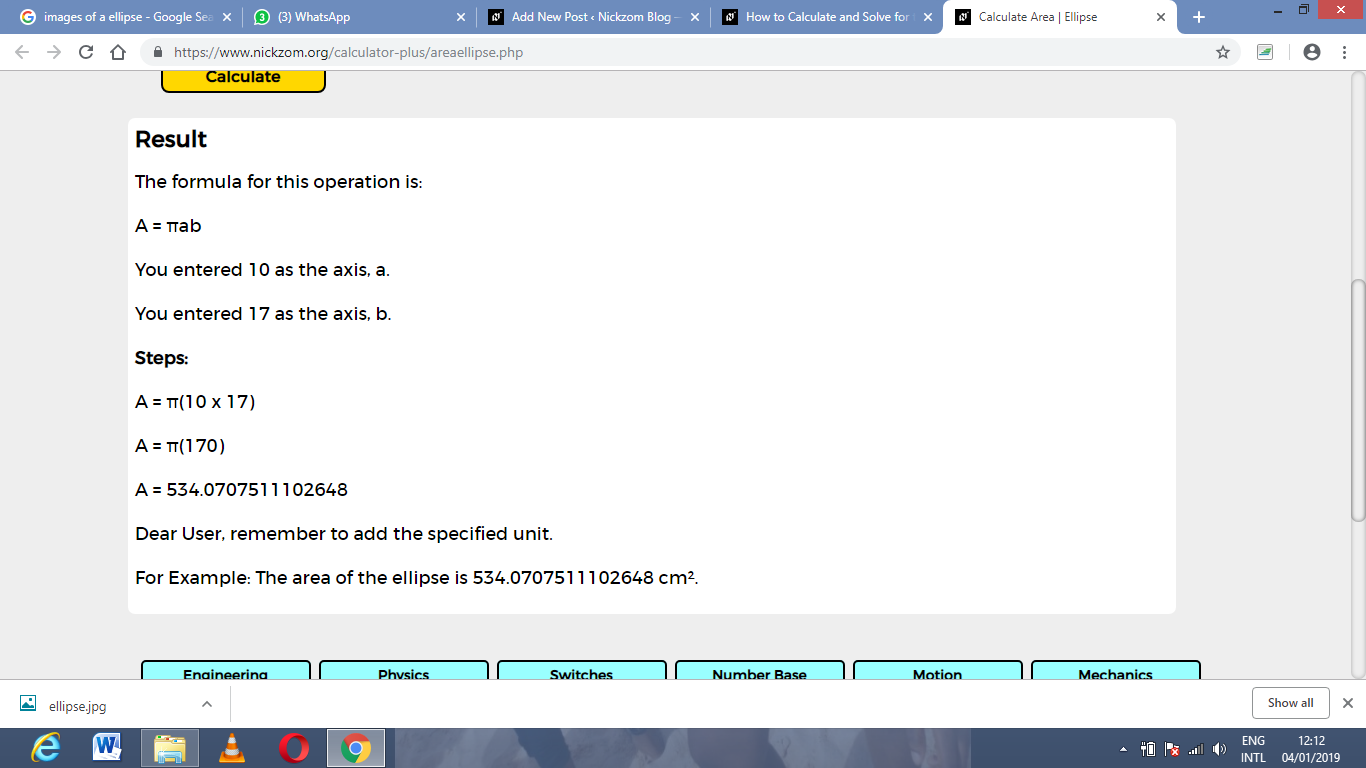As you can see from the screenshot above, Nickzom Calculator – The Calculator Encyclopedia solves for the area of an ellipse and presents the formula, workings and steps too.